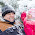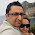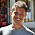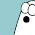## Saturday, 5 May 2012

### Introduction

.NET has moved to become more of a functional programming since .NET 3.0. Currently there are many ways that you can invoke execution of code such as direct call, reflection, delegates, compiled expressions, dynamic, etc. In this post I will compare performance of these methods. Part of the inspiration for this comparison was reviewing the ASP.NET Web API code.

### Readability/maintainability vs. Performance

Functional programming is on the rising popularity. Why now, is it not decades old?

Part of the popularity goes back to the fact that now we run much faster machines - with more cores. And we need to solve more complex problems and there is a need to write more readable code and be able to expression the code more succinctly. On the top of all this, add the fact that we need to invoke code in parallel to get the best of our machines horse power.

We are writing code that compared to 20 years ago is quite inefficient, using more processor cycles. C was slower than Assembly, and C# slower than C but it certainly does not make sense to write in Assembly. But language is only part of the story. We get choices in one language itself. In .NET, we sometimes use IEnumerable<T>.Count() instead of using IList<T>.Count. While .NET in case of .Count() first tries to convert the IEnumerable<T> to ICollection<T> and if it does not succeed then loops through to get the count, in other cases framework cannot improve the performance.

Our code is commonly a compromise between readability and performance. I for one would pick former over latter but my decision needs to be an informed decision. If a code is 1000 times slower (and that part of the code is called many times) I definitely would sacrifice readability, but if it is only twice slower, I would pick readability.

Context is also important. Poor performance on server can be costly but on the client usually would not be noticed. A real-time image processing code has to squeeze every drop of performance it can (having done real-time image processing in C++, I am pretty familiar with it), while an asynchronous batch process could use a more liberal approach. In any case, micro-optimisation is a common pitfall that I try not to fall into.

### Code Invocation

In .NET we can use different ways to invoke some code (colour coded based on category):
1. Static method call
2. Instance method call
3. Instance method call on virtual methods where CLR has to walk up/down the inheritance hierarchy to understand the piece of code to run.
4. Invocation using reflection
5. Invocation using a previously bound reflected object
6. Invoking a delegate
7. Invoking a Func or a compiled expression (which itself is a delegate)
8. DynamicInvoke on a delegate
9. Compiling a lambda expression and executing it
10. Invocation on a dynamic object
For our test, I am using a simple class which calculates tangent of an angle. I have done all I could to make sure condition for all these scenarios are similar so that the difference in performance is only related to the call method.
```internal class WidgetBase
{
public virtual double VirtualGetTangent(double value)
{
throw new NotSupportedException();
}
}

internal class Widget : WidgetBase
{

public override double VirtualGetTangent(double value)
{
return Math.Sin(value) / (Math.Cos(value) + 0.0000001f);
}

public virtual double InstanceGetTangent(double value)
{
return Math.Sin(value) / (Math.Cos(value) + 0.0000001f);
}

public static double GetTangent(double value)
{
return Math.Sin(value) / (Math.Cos(value) + 0.0000001f);
}

}
```

Now let's see how each scenario is implemented (I have commonly ignored the return value).
```static void CallStatic(double value)
{
Widget.GetTangent(value);
}

static void CallInstance(double value, Widget widget)
{
widget.InstanceGetTangent(value);
}

static void CallVirtualInstance(double value, Widget widget)
{
widget.VirtualGetTangent(value);
}
```

First three call types need not much explanation. Static method and instance method do not have much difference. Internally in CLR, instance method has an additional parameter for the instance itself passing this to it. Jeff Richter explains the difference between IL's call and callvirt where callvirt is slower than call but as we will see difference is minimal.
```static void CallReflector(double value, Widget widget)
{
MethodInfo methodInfo = typeof(Widget).GetMethods(BindingFlags.Instance |
BindingFlags.Public).Where(x => x.Name == "InstanceGetTangent").First();
methodInfo.Invoke(widget, new object[] { value });
}

static void CallMethodInfo(double value, MethodInfo methodInfo, Widget widget)
{
methodInfo.Invoke(widget, new object[] { value });
}
```
With reflection, we have two steps. First one is binding where we get the MethodInfo from the type object. Next one is the actual execution where we use Invoke to execute the method. As you can see, second method is passed the MethodInfo while the first one binds every time. In our example, this will incur the overhead of boxing since both our input and output are double while parameters passed in and out of Invoke is object. However, I have decided to keep it so since this could also happen in a real scenario.

```public delegate double CalculateIt(double value);

static void CallDelegate(double value, CalculateIt d)
{
d(value);
}

static void CallFunc(double value, Func<double, double> func)
{
func(value);
}

static void CallDelegateDynamicInvoke(double value, Delegate d)
{
d.DynamicInvoke(new object[] { value });
}

static void CallExpression(double value, Expression<Func<double, double>> expression)
{
Func<double , double> compile = expression.Compile();
compile(value);
}
```
CalculateIt is a delegate defined above and called in the first method. Difference between first call and third is that the first one is a strongly typed delegated while the third one is simply weakly typed delegate that can be only called using DynamicInvoke.
```static void CallDynamic(double value, Widget widget)
{
dynamic d = widget;
d.InstanceGetTangent(value);
}```
Dynamic object call is simple and uncomplicated as can be seen above.

```NOTE: Metrics of running a compiled expression is the same as that of Func so it is not separately calculated. My point here is to show that compiling an expression all the time is expensive and I have seen cases were people do it - if you have not seen. I particularly saw an example where it was used for Null Guard expressions.
```

### Test execution

Each method was called 10,000,000 times and results where compared. I have used a code which is very verbose but I was trying to eliminate anything that could skew the results.

There are other factors such as garbage collection and other processes running on the machine but I have ran the code quite a few times and results were more or less consistent with only 1-2% variation.

### Results review

As it can be seen, compiling an expression is 10 times slower than most other call types including Func<T> delegates (result of expression compilation). This is particularly important since some of the new frameworks (e.g. ASP.NET Web API) heavily use expression compilation.
Also of importance is that binding is significant overhead in reflection. By binding once and invoking many times we can improve the performance.

Another important insight is that dynamic, unlike what it is famous for, is not that much slower than strongly typed direct calls (less than twice).

### Conclusion

Compiling a lambda expression and running it is the slowest type of code invocation. There is not a meaningful difference between calling a static, instance, virtual method or Func/delegate. Calling a method on a dynamic object less than twice slower than making a direct call.

### Source code

As I said, my code is very verbose to eliminate any element that could skew the result. In case you need to review, modify or run the source code, I have brought the source code here:

```using System;
using System.Collections.Generic;
using System.Diagnostics;
using System.Dynamic;
using System.IO;
using System.Linq;
using System.Linq.Expressions;
using System.Reflection;
using System.Runtime.InteropServices;
using System.Security.AccessControl;
using System.Text;
using System.Threading;

namespace PerformanceComparison
{

class Program
{
private static Random _random = new Random();
public delegate double CalculateIt(double value);

static void Main(string[] args)
{

const int TotalCount = 10000 * 1000; // 10 million
Stopwatch stopwatch = new Stopwatch();
Expression<Func<double, double>> expression =
(double value) => Math.Sin(value) / (Math.Cos(value) + 0.0000001f);
Func<double, double> func = expression.Compile();
Widget widget = new Widget();

Thread.Sleep(2000);

// ________   Static ____________________________________________________
Console.WriteLine("Static ---------------------------------------");
stopwatch.Start();
for (int i = 0; i < TotalCount; i++)
{
CallStatic(_random.NextDouble());
}
stopwatch.Stop();
Console.WriteLine(stopwatch.Elapsed.ToString());
stopwatch.Reset();

// ________   Instance ____________________________________________________
Console.WriteLine("Instance ---------------------------------------");
stopwatch.Start();
for (int i = 0; i < TotalCount; i++)
{
CallInstance(_random.NextDouble(), widget);
}
stopwatch.Stop();
Console.WriteLine(stopwatch.Elapsed.ToString());
stopwatch.Reset();

// ________   Instance Virtual _______________________________________________
Console.WriteLine("Instance Virtual --------------------------------");
stopwatch.Start();
for (int i = 0; i < TotalCount; i++)
{
CallVirtualInstance(_random.NextDouble(), widget);
}
stopwatch.Stop();
Console.WriteLine(stopwatch.Elapsed.ToString());
stopwatch.Reset();

// ________   Reflected ____________________________________________________
Console.WriteLine("Reflected ---------------------------------------");
stopwatch.Start();
for (int i = 0; i < TotalCount; i++)
{
CallReflector(_random.NextDouble(), widget);
}
stopwatch.Stop();
Console.WriteLine(stopwatch.Elapsed.ToString());
stopwatch.Reset();

// ________   Reflected after binding ___________________________________________________
Console.WriteLine("Reflected after binding ------------------------------");
MethodInfo methodInfoInstance = typeof(Widget).GetMethod("InstanceGetTangent",
BindingFlags.Instance | BindingFlags.Public);
stopwatch.Start();
for (int i = 0; i < TotalCount; i++)
{
CallMethodInfo(_random.NextDouble(), methodInfoInstance, widget);
}
stopwatch.Stop();
Console.WriteLine(stopwatch.Elapsed.ToString());
stopwatch.Reset();

// ________   Delegate ____________________________________________________
Console.WriteLine("Delegate -------------------------------------------------");
stopwatch.Start();
CalculateIt c = widget.InstanceGetTangent;
for (int i = 0; i < TotalCount; i++)
{
CallDelegate(_random.NextDouble(), c);
}
stopwatch.Stop();
Console.WriteLine(stopwatch.Elapsed.ToString());
stopwatch.Reset();

// ________   Func ____________________________________________________
Console.WriteLine("Func -------------------------------------------------");
stopwatch.Start();
for (int i = 0; i < TotalCount; i++)
{
CallFunc(_random.NextDouble(), func);
}
stopwatch.Stop();
Console.WriteLine(stopwatch.Elapsed.ToString());
stopwatch.Reset();

// ________   Delegate (Dynamic Invoke) ____________________________________________________
Console.WriteLine("Delegate DynamicInvoke ------------------------------------------");
stopwatch.Start();
for (int i = 0; i < TotalCount; i++)
{
CallDelegateDynamicInvoke(_random.NextDouble(), func);
}
stopwatch.Stop();
Console.WriteLine(stopwatch.Elapsed.ToString());
stopwatch.Reset();

// ________   Expression ____________________________________________________
Console.WriteLine("Expression -------------------------------------------------");
stopwatch.Start();
for (int i = 0; i < TotalCount / 100; i++)
{
CallExpression(_random.NextDouble(), expression);
}
stopwatch.Stop();
Console.WriteLine(stopwatch.Elapsed.ToString());
stopwatch.Reset();

// ________   Dynamic ____________________________________________________
Console.WriteLine("Dynamic -------------------------------------------------");
stopwatch.Start();
for (int i = 0; i < TotalCount; i++)
{
CallDynamic(_random.NextDouble(), widget);
}
stopwatch.Stop();
Console.WriteLine(stopwatch.Elapsed.ToString());
stopwatch.Reset();

Console.Read();
}

static void CallStatic(double value)
{
Widget.GetTangent(value);
}

static void CallInstance(double value, Widget widget)
{
widget.InstanceGetTangent(value);
}

static void CallVirtualInstance(double value, Widget widget)
{
widget.VirtualGetTangent(value);
}

static void CallReflector(double value, Widget widget)
{
MethodInfo methodInfo = typeof(Widget).GetMethod("InstanceGetTangent",
BindingFlags.Instance | BindingFlags.Public);
methodInfo.Invoke(widget, new object[] { value });
}

static void CallMethodInfo(double value, MethodInfo methodInfo, Widget widget)
{
methodInfo.Invoke(widget, new object[] { value });
}

static void CallDelegate(double value, CalculateIt d)
{
d(value);
}

static void CallFunc(double value, Func<double, double> func)
{
func(value);
}

static void CallDelegateDynamicInvoke(double value, Delegate d)
{
d.DynamicInvoke(new object[] { value });
}

static void CallExpression(double value, Expression<Func<double, double>> expression)
{
Func<double, double> compile = expression.Compile();
compile(value);
}

static void CallDynamic(double value, Widget widget)
{
dynamic d = widget;
d.InstanceGetTangent(value);
}

}

internal class WidgetBase
{
public virtual double VirtualGetTangent(double value)
{
throw new NotSupportedException();
}
}

internal class Widget : WidgetBase
{

public override double VirtualGetTangent(double value)
{
return Math.Sin(value) / (Math.Cos(value) + 0.0000001f);
}

public virtual double InstanceGetTangent(double value)
{
return Math.Sin(value) / (Math.Cos(value) + 0.0000001f);
}

public static double GetTangent(double value)
{
return Math.Sin(value) / (Math.Cos(value) + 0.0000001f);
}

}

}
```

#### 6 comments:

1.Why use compile several million times? No one do this. Need to call non compiled expression, or use compiled cached version. Those two types of use are most common.

1.Usually you wouldn't. The point of the article is to compare the overhead in terms of orders of magnitude.

Yet, there are cases where you might do this especially when you dynamically compose an expression tree or when loading an expression tree from its persisted state. Although you might be able to cache based on the hash of the persisted stream, it is important to quantify the overhead.

2.Try this:

// ________ Compiled ____________________________________________________
Console.WriteLine("Compiled -------------------------------------------------");
stopwatch.Start();
Compile(expression);
for (int i = 0; i < TotalCount / 100; i++) {
CallCompiled(_random.NextDouble());
}
stopwatch.Stop();
Console.WriteLine(stopwatch.Elapsed.ToString());
stopwatch.Reset();

...

static Func compiled = null;
private static void Compile(Expression> expression) {
compiled = expression.Compile();
}
private static void CallCompiled(double value) {
compiled(value);
}

1.I have already said that the metrics of running a compiled expression is the same as of a Func.

3.Can you tell me more about your web-blog system? Your website is attractive and is designed well. I like to write and have tried out my luck at a few marketplaces such as freelance advertising jobs, online video uploading sites and www.odesk.com. I must say that I did make money. Your ideas are quite interesting and well-written. Kudos! Waiting for more such blogs.

4.Compiling the expression inside the loop is ridiculous. Of course it will be slow as hell. That's the whole point of compiling: to spend some extra time *once*, in order to get benefits later. That's exactly why the "func" test is faster that plain delegate invocation (poor name for the test, btw, since `Func` is a generic delegate also, it would make much more sense to name this test "precompiled expression" instead).

Note: only a member of this blog may post a comment.